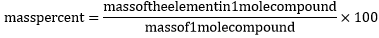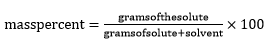# Mass Percent Formula

## Mass Percent Formulas - Examples & Practice Questions

Mass percent is another method of expressing the composition of the solution mixture. It describes the amount of solute (grams) present in 100 gm of the solution. Let us look at the to two commonly used formula to find the mass percentage in a given question.

Case I:
You are asked to find out the mass percentage of an element in a compound, the formula used will be:Case II:
The Formula for mass of a element/compound in a solution/mixtureExample: Find the mass percentage of 6 g sodium hydroxide dissolved in 50 g of water. (Note: since the density of water is nearly 1, this type of question often gives the volume of water in millilitres.)
Solution:
First find the total mass of the solution:
total mass = 6 g sodium hydroxide + 50 g water total mass = 56 g
Now, you can find the mass percentage of the sodium hydroxide using the formula:
mass percent = (grams of solute / grams of solution) x 100 mass percent = (6 g NaOH / 56 g solution) x 100 mass percent = (0.1074) x 100 = 10.74% NaOH

Question: Find the masses of sodium chloride and water required to obtain 175 g of a 15% solution.
Options:
(a) NaCl = 26.25 gm; water = 147.75 g
(b) NaCl = 36.25 gm; water = 147.75 g
(c) NaCl = 26.25 gm; water = 167.75 g
(d) NaCl = 40.25 gm; water = 147.75 g